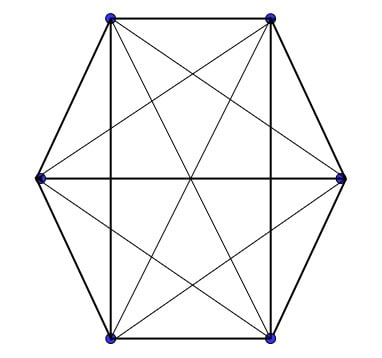## 1. Introduction

In this tutorial, we’ll talk about the concepts of the Ramsey theorem. We’ll present the advantages and everyday use cases of Ramsey’s theory.

## 2. What’s the Ramsey Theory?

Ramsey theory is a branch of mathematics that focuses on the study of combinatorial objects in which some degree of order must occur as the size of the objects grows. In other words, Ramsey’s theory studies the following problem: Given a compositional structure (integer or subset of a graph), how large must that structure be to guarantee the existence of a substructure (subset or subgraph) of a particular property?

The theory was introduced by Frank Plumpton Ramsey and had many applications in communication network design.

## 3. Ramsey’s Theorem

The most famous example of Ramsey’s theory is Ramsey’s theorem, which generalizes the following puzzle.

Example: Prove that there are a group of three shared friends or a group of three mutual non-friends at any party with at least six people.

Solution: Name the people,,,,, and. Either A has three friends or three, not friends. Without loss of generality, let’s assume that,, andare all friends of. Then, if a pair of them are friends, the pair plusforms a set of three mutual friends. Else, the two of them are a set of three mutual non-friends.

Theorem: A monochromatic complete subgraph can be found if the edges of a sufficiently sizeable full graph are coloured in any way.

The Ramsey theorem has some mathematical concepts: friends and strangers, two colours, and infinite case theorems.

### 3.1. Ramsey’s Theorem: Friends and Strangers

Consider a group of six people. Let’s consider two of them. Whether they’re meeting for the first time — in which case we refer to them as mutual strangers—or have already met, we refer to them as mutual acquaintances. According to the theorem, each group of six people consisted of at least three pairs of strangers or acquaintances who knew each other.

A Dinner Party Problem. Let’s say a dinner party for six people. Then there is a trio of guests at the party who are either acquainted or utterly unrelated. The following figure presents the dinner party problem:### 3.2. Ramsey’s Theorem: Ramsey Number, Two Colors

Ramsey’s theorem reformulates as follows: Fix positive integers. Any complete graph with enough vertices such that every edge is red or blue will contain either a blue clique of n vertices or a red clique of m vertices.

The Ramsey numberis the minimum party size that guarantees a set of n mutual non-friends or m mutual friends. Or, given a blue clique of n vertices or a red clique of m vertices, the minimum number of vertices the complete graph must have for each edge to be coloured red or blue.

For two colours, Ramsey’s theorem states that for every pair of positive integersthere is the smallest positive integer. Consequently, for every complete graph on vertices, the edges are coloured blue or red. So that, either the complete subgraph with n vertices is completely blue, or the complete subgraph with m vertices is completely red.

### 3.3. Ramsey’s Theorem: Infinite Case

The theorem generalizes to any (finite) number of colours; there is a Ramsey numberthat guarantees that in sufficiently large graphs, there aremonochromatic cliques with vertices of colour.

Let’s introduce an example:is trivial.  (No need for colour in cliques with a single vertex.)Since ineither all edges are red, there is a red blob at m vertices, or there is a blue edge somewhere. In this case, the two vertices it connects are the blue blobs on both vertices. In other words, either everyone at the party is friends with everyone else, or two people are not friends.This is same example as in previous section.

## 4. Applications of Ramsey Theory

Ramsey’s theory has many interesting applications, including results in numbers, geometry, algebra, topology, logic, set theory, ergodic theory, theoretical computer science, and information theory. These applications come from the fields of communication, decision-making, and information retrieval in computer science.

However, Ramsey’s theory demonstrates this in specific applications such as optical channel coding, electricity pricing, and, more recently, financial distortions.

## 5. Conclusion

According to Ramsey’s theory, there are general prerequisites for the existence of substructures with regular qualities. In this application, it is a problem of the presence of monochromatic subsets or subsets of connected vertices of only one colour. Ramsey’s theory finds applications in many domains like communication and finance.

1 Comment
Inline Feedbacks